# Generalized Linear Models

Benedikt Ehinger
March 2017

### How to use these slides

this is a link, it will be green

print('this is some r code')

 "this is some r output"


### Differencs to Basil

• Metric <-> Continuous
• $$\beta$$ -> I use it for standardized and non-standardized predictors (sorry :-)

### Overview

• Binary data
• Example
• Logistic regression
• Interpretation of coefficients
• Checking the model
• Inference
• Poisson Regression
• GLMs
• Assumptions

### Overview

• Binary data
• Example
• Logistic regression
• Interpretation of coefficients
• Checking the model
• Inference
• Poisson Regression
• GLMs
• Assumptions

### Example tasks with binomial dataIs it the same array?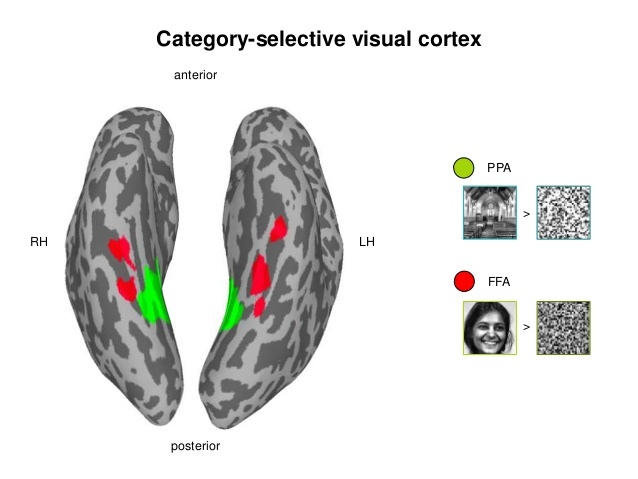Decoding: Is it a face or a house? Based on BOLD/EEG data

Will the patient survive?Did you see a grating?

### Contrast Detection Task

• Task: Do you observe a grating?
• Research Question: What is the minimum contrast to reliably (>25%) detect a grating?n.trial =150
n.contrastLevels=12


### The Data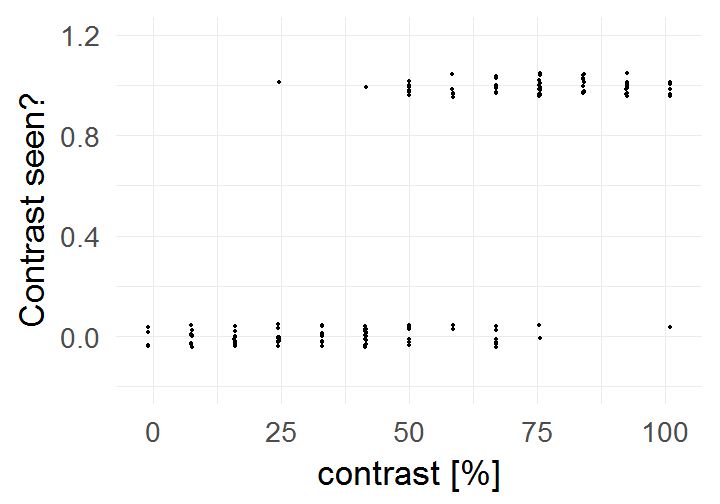### What is the probability to detect the target?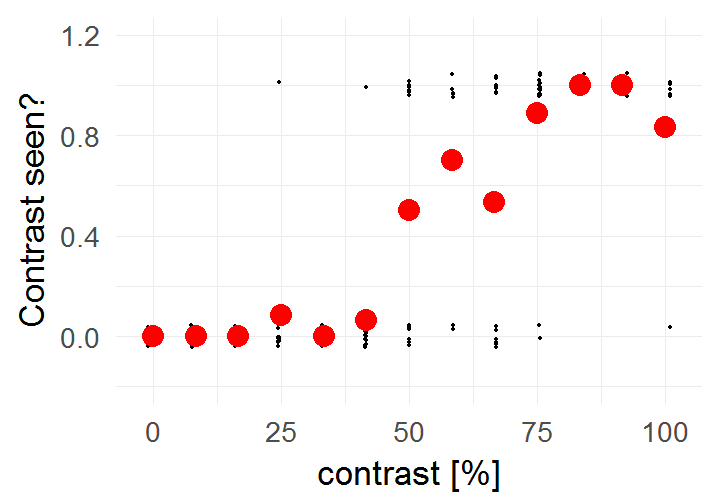### Let's try linear regression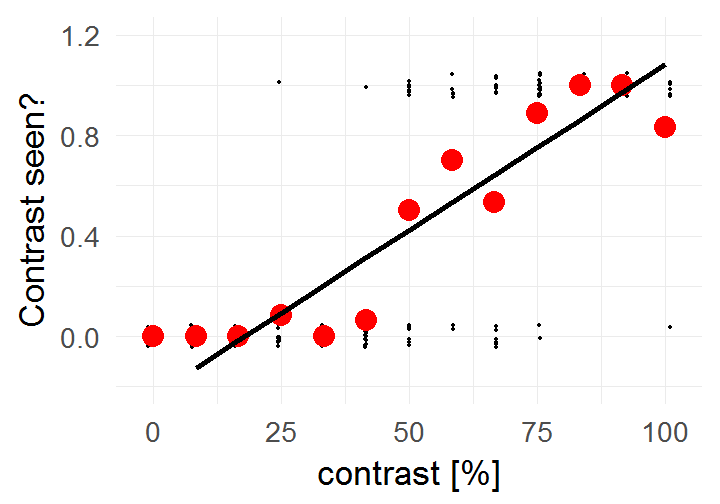There are no negative probabilities. What to do?

### Truncating everything smaller 0 / larger 1: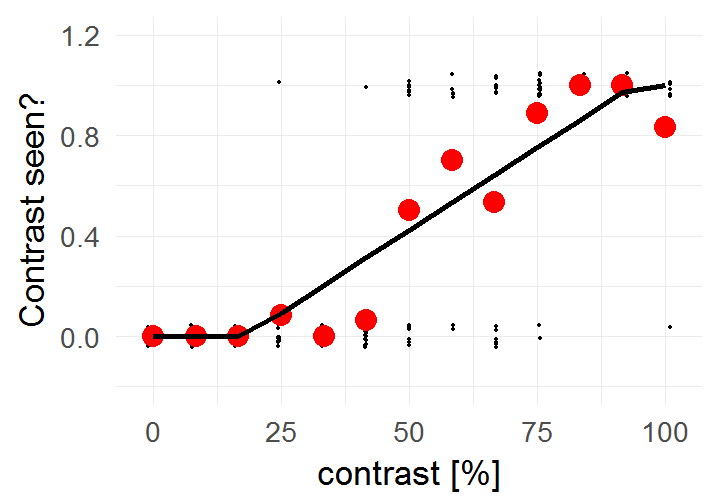A contrast of ~16% will be rejected in 100% of cases.

A strong statement!

### A better fit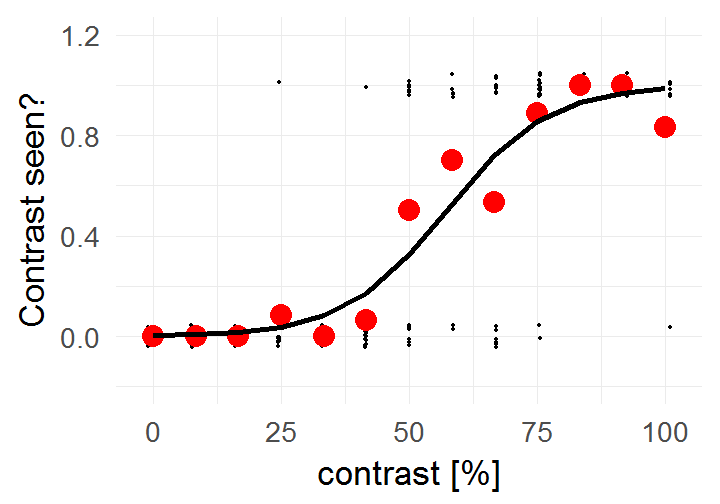### How to define differences in probabilities

$p(coxi==nerd) = 0.75)$ $p(psycho==nerd) = 0.6)$

Risk Difference: 0.75 - 0.6 => coxis are 15 percentage points more likely to be nerds

Relative Risk: $$\frac{0.75}{0.6} = 1.25$$ => Coxis are 25% more likely to be nerds

Relative Risks are intuitive but non symmetric:

$$\frac{p(X)}{p(Y)} = \frac{0.99}{0.98} = 1.01$$ => X is 1% more likely than Y

$$\frac{p(\neg X)}{p(\neg Y)} = \frac{0.01}{0.02} = 0.5$$ => not X is 50% more likely than not Y

### The problem with relative risks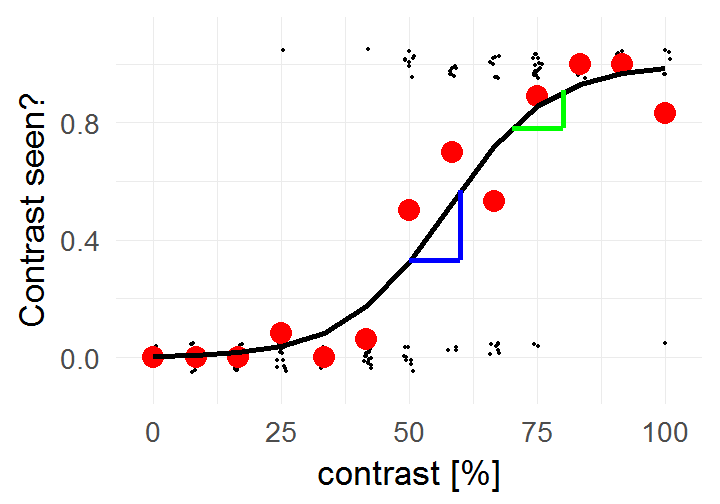p(X|contrast=50) / p(X|contrast=60) = 0.33/0.57 = 0.58

p(X|contrast=70) / p(X|contrast=80) = 0.78/0.91 = 0.86

An increase of 10 on X is not a constant increase in the relative risks.

### How to get an increase of 10 in X an linear increase in y?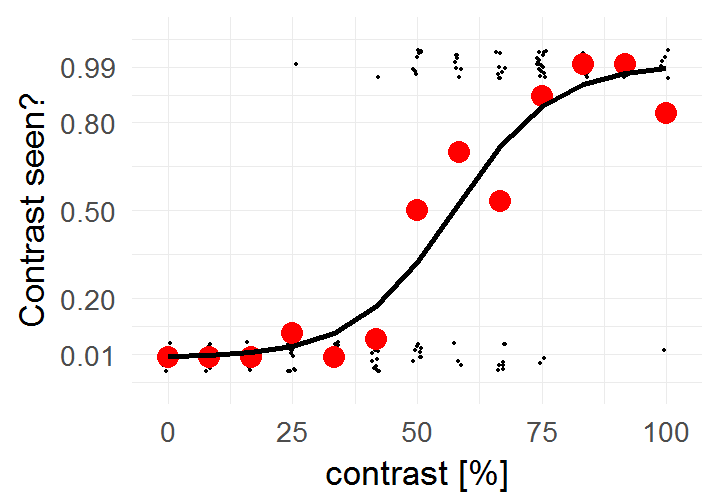### transforming y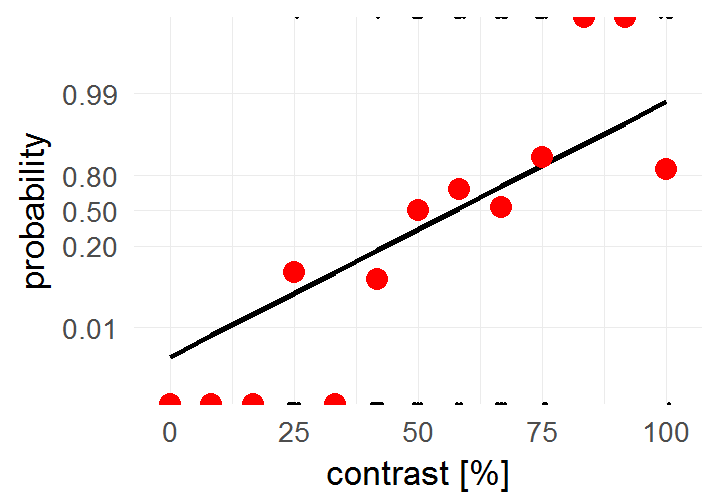An alternative view, same data but different y-axis

### logit & inv.logit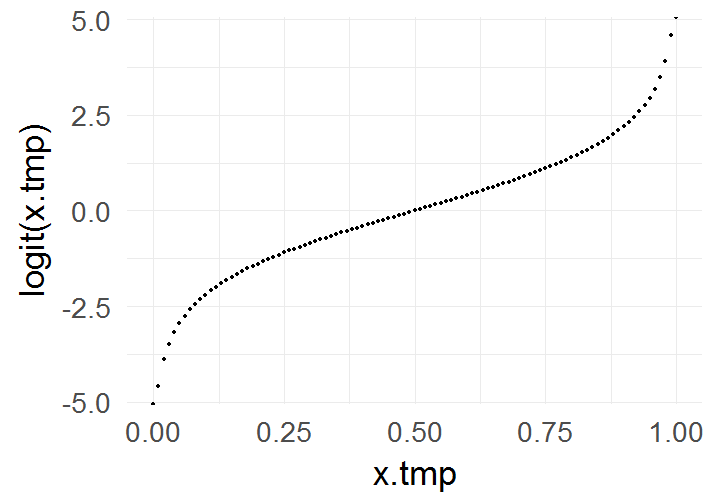From $$[0, 1]$$ to $$[-\infty, +\infty]$$

$x = log(\frac{p}{1-p})$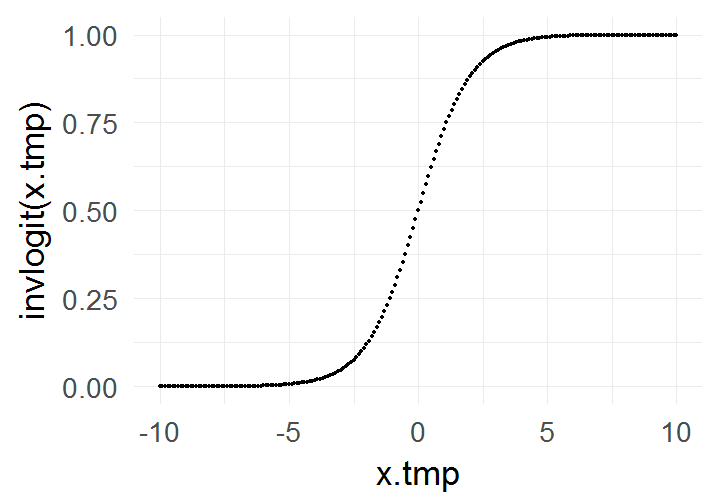From $$[-\infty, +\infty]$$ to $$[0, 1]$$

$p = \frac{1}{1+e^{-x}}$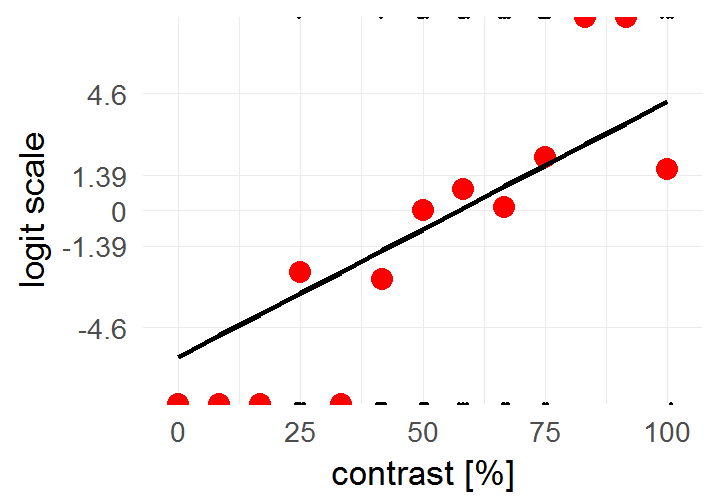The domain we are working in goes from $$-\infty$$ to $$+\infty$$. We hope our relationships are additive in this domain

### Overview

• Binary data
• Example
• Logistic regression
• Interpretation of coefficients
• Checking the model
• Inference
• Poisson Regression
• GLMs
• Assumptions

### logistic regression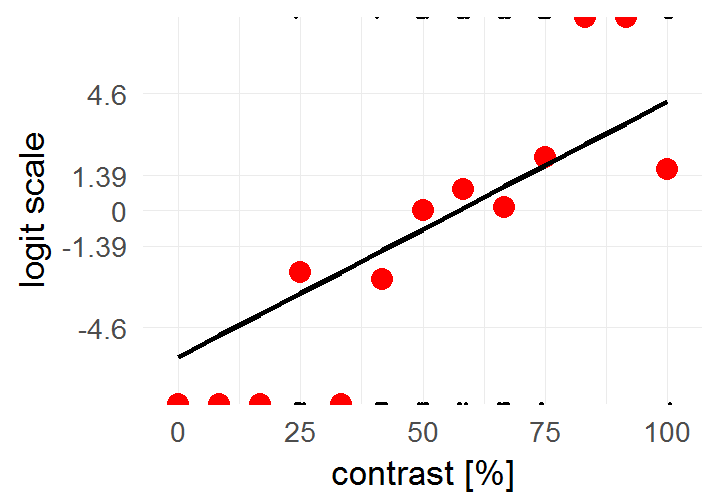$$y_i = invlogit(\beta X + e_i)$$ <=> $$logit(y_i) = \beta X + e_i$$

$y_i = g(\beta X + e_i)$

yes! that is the g from GLM

We call this logistic regression

### the interplay of our two predictors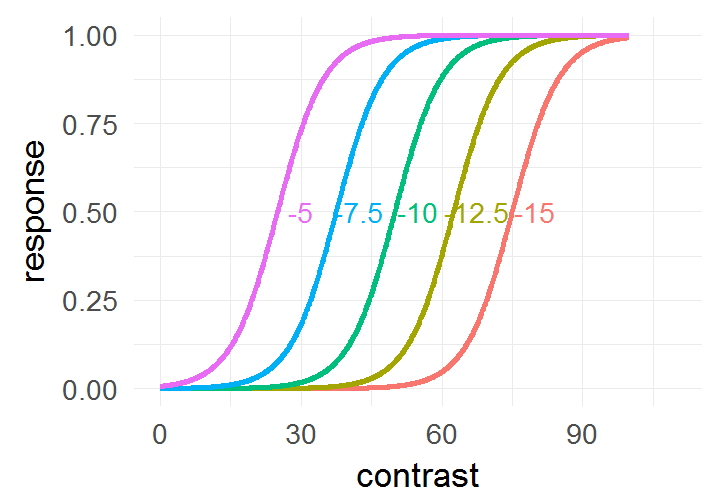Changing Intercept $$\beta_0$$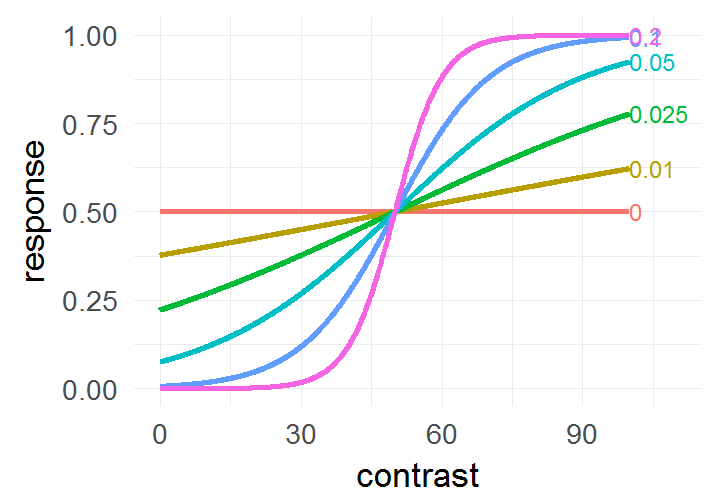Changing Slope $$\beta_1$$

### What are the units on the y-axis?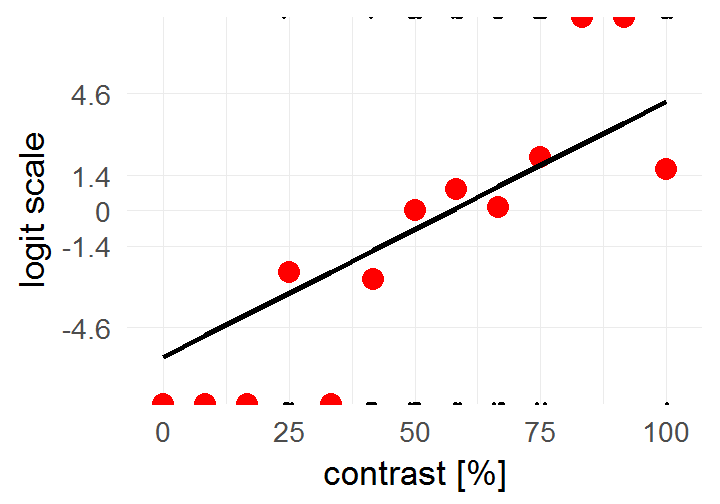$invlogit = \frac 1 {1+e^{-x}}$

$logit = log(\frac p {1-p}) = log(odds)$

What are odds?

### What are odds?

Odds are a somewhat unintuitive quantity: $p(coxi==nerd) = 0.75$

$odds(coxi==nerd) = \frac{p(coxi==nerd)}{p(coxi \neq nerd)} = \frac{0.75}{1-0.75} = \frac{0.75}{0.25} = 3$

In words: the odds for an cogsci to be a nerd are 3 to 1

For 3 nerd coxis you will find 1 non-nerd

### What are odds?

$odds = \frac{p}{1-p}$ ($$odds \in [0,+\infty]$$, halfway there)

taking the log()

$log(odds) = logit = log(\frac{p}{1-p})$

This is a mapping from $$p \in [0,1]$$ to $$logit(p) \in [-\infty +\infty]$$

### Summary

$y_i = g(\beta X + e_i)$

$$y_i = invlogit(\beta X + e_i)$$ <=> $$logit(y_i) = \beta X + e_i$$

• Binary data (0/1, True/False)
• Units are log(odds)
• one of many link-functions of GLMs
• It is possible to use other functions that map $$p \in [0,1]$$ to $$logit(p) \in [-\infty +\infty]$$, e.g. probit (cumulative normal). But the units are not as easily interpretable as odds (!)

### Running a logistic regression in R


Call:
glm(formula = response ~ 1 + contrast, family = binomial, data = d)

Deviance Residuals:
Min       1Q   Median       3Q      Max
-2.9298  -0.4171  -0.1217   0.5597   2.5579

Coefficients:
Estimate Std. Error z value Pr(>|z|)
(Intercept) -5.73617    0.91880  -6.243 4.29e-10 ***
contrast     0.10014    0.01556   6.437 1.22e-10 ***
---
Signif. codes:  0 '***' 0.001 '**' 0.01 '*' 0.05 '.' 0.1 ' ' 1

(Dispersion parameter for binomial family taken to be 1)

Null deviance: 205.78  on 149  degrees of freedom
Residual deviance: 103.39  on 148  degrees of freedom
AIC: 107.39

Number of Fisher Scoring iterations: 6


### Overview

• Binary data
• Example
• Logistic regression
• Interpretation of coefficients
• Checking the model
• Inference
• Poisson Regression
• GLMs
• Assumptions

### The constant

TLDR; $$\beta_0$$ = log-odds if everything else is 0.

$$\beta_0$$<0 then p<0.5

$$\beta_0$$==0 then p=0.5

$$\beta_0$$>0 then p>0.5

$y(x) = invlogit(\beta_0 + \beta_1 x)$

$y(x) = \frac{1}{1 + e^{-(\beta_0 + \beta_1 x)}}$

$y(x=0) = \frac{1}{1 + e^{-\beta_0}}$

### In our example

coef(glm(response~contrast,data=d,family=binomial)) # log-odds

(Intercept)
-5.736166

invlogit(coef(glm(response~contrast,data=d,family=binomial))) # probability

(Intercept)
0.003216738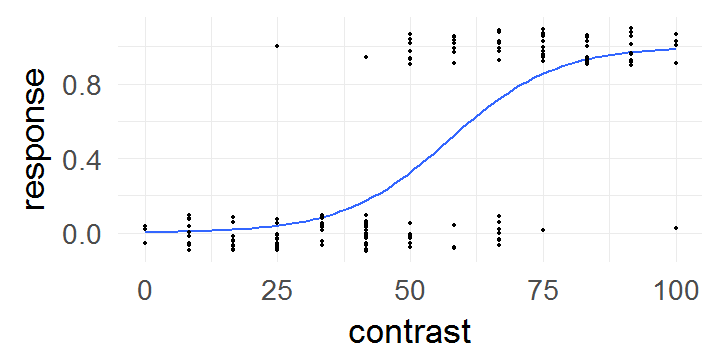### Interpreting the slope

TLDR; $$\beta_1$$ = how much x increases changes the log(odds-ratio)

$log(odds) = logit(\hat y(x)) = \beta_0 + \beta_1 x$
An increase by 1 x $$\beta_1$$:

$logit(\hat y(x+1)) = \beta_0 + \beta_1 (x+1)$
Taking the difference:

$logit(\hat y(x)) - logit(\hat y(x+1)) = \beta_0 + \beta_1 x -\beta_0 + \beta_1 (x+1) = \beta_1$
Using the log-rule on the left side ($$log(a) - log(b) = log(\frac a b )$$)

$logit(\hat y(x) / \hat y(x+1)) = \beta_1$
$\beta_1 = log(\frac{\hat y(x) / \hat y(x+1)}{1-\hat y(x) / \hat y(x+1)})$
$$e^{\beta_1} =$$ odds-ratio$$_{\hat y(x)/\hat y(x+1)}$$

### What are odds-ratios?

$odds(coxi==nerd) = 3$

$odds(psycho==nerd) = 1.5$

$odds-ratio = \frac{3}{1.5} = 2$

The odds that coxis are nerds are 2x as high than the odds that psychology students are nerds.

(remember relative risk: Coxis are 1.25 as likely than psychology students to be nerds)

$e^{\beta_1} = odds-ratio( y(x)/y(x+1))$

The odds that you will succeed are $$e^{beta}$$ higher at $$y(x+1)$$ than $$y(x)$$

### Back to the example

co = coef(glm(response~contrast,data=d,family=binomial)) # log-odds ratio
co["contrast"]

 contrast
0.1001421

exp(co["contrast"]) # odds ratio

contrast
1.105328


With a step of 1, the log-odds change by ~1.1

### Relative Risk

What is the relative risk between contrast = 50 and contrast = 80?

a = invlogit(co["(Intercept)"]+co["contrast"] * 80)
b = invlogit(co["(Intercept)"]+co["contrast"] * 50)
unname(a)

 0.9068021

unname(b)

 0.3254005

unname(a/b)

 2.786726


we have ~280% more successes at contrast = 80 than at contrast = 50

### Overview

• Binary data
• Example
• Logistic regression
• Interpretation of coefficients
• Checking the model
• Inference
• Poisson Regression
• GLMs
• Assumptions

### Residuals


Call:
glm(formula = response ~ 1 + contrast, family = binomial, data = d)

Deviance Residuals:
Min       1Q   Median       3Q      Max
-2.9298  -0.4171  -0.1217   0.5597   2.5579

Coefficients:
Estimate Std. Error z value Pr(>|z|)
(Intercept) -5.73617    0.91880  -6.243 4.29e-10 ***
contrast     0.10014    0.01556   6.437 1.22e-10 ***
---
Signif. codes:  0 '***' 0.001 '**' 0.01 '*' 0.05 '.' 0.1 ' ' 1

(Dispersion parameter for binomial family taken to be 1)

Null deviance: 205.78  on 149  degrees of freedom
Residual deviance: 103.39  on 148  degrees of freedom
AIC: 107.39

Number of Fisher Scoring iterations: 6


### Deviance / Residuals

$$\hat{y}_i = invlogit(\beta X)$$

$$LL(y_1|\hat y_i) =$$ loglikelihood $$=log(\prod{\hat{y_i}^{y_i} (1-\hat{y_i})^{(1-y_i)}})$$

for a single data point simplifies to

if $$y_i = 1$$:    $$log(\hat{y_i})$$      if $$y_i = 0$$:   $$log(1-\hat{y_i})$$

Deviance Residuals: $$d_i = s_i\sqrt{-2LL(y_i|\hat y_i)}$$ $$s_i = 1$$ if $$y_i = 1$$, $$s_i = -1$$ if $$y_1 = 0$$

Intuition words: deviance is a measure of how well the model fits a data point.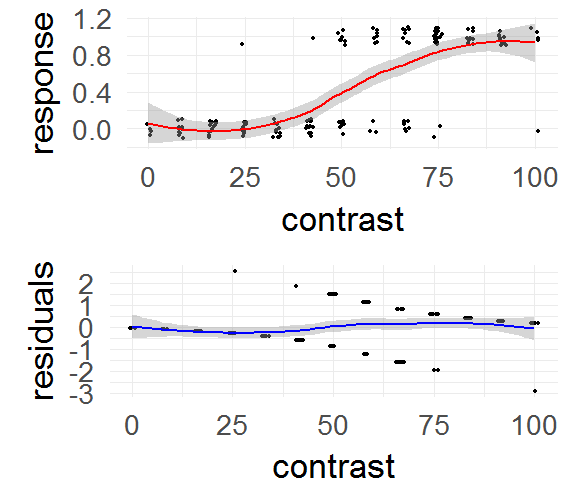### Null & Residual Deviance


Call:
glm(formula = response ~ 1 + contrast, family = binomial, data = d)

Deviance Residuals:
Min       1Q   Median       3Q      Max
-2.9298  -0.4171  -0.1217   0.5597   2.5579

Coefficients:
Estimate Std. Error z value Pr(>|z|)
(Intercept) -5.73617    0.91880  -6.243 4.29e-10 ***
contrast     0.10014    0.01556   6.437 1.22e-10 ***
---
Signif. codes:  0 '***' 0.001 '**' 0.01 '*' 0.05 '.' 0.1 ' ' 1

(Dispersion parameter for binomial family taken to be 1)

Null deviance: 205.78  on 149  degrees of freedom
Residual deviance: 103.39  on 148  degrees of freedom
AIC: 107.39

Number of Fisher Scoring iterations: 6


Null Deviance$$^2$$: $$2(LL(M_{saturated}) - LL(M_0))$$

Residual Deviance$$^2$$: $$2(LL(M_{saturated}) - LL(M_1))$$

### Overview

• Binary data
• Example
• Logistic regression
• Interpretation of coefficients
• Checking the model
• Inference
• Poisson Regression
• GLMs
• Assumptions

### Model comparison

m0 = glm(response~1           ,data=d,family=binomial)
m1 = glm(response~1 + contrast,data=d,family=binomial)
anova(m0,m1,test='LRT')

Analysis of Deviance Table

Model 1: response ~ 1
Model 2: response ~ 1 + contrast
Resid. Df Resid. Dev Df Deviance  Pr(>Chi)
1       149     205.78
2       148     103.39  1   102.39 < 2.2e-16 ***
---
Signif. codes:  0 '***' 0.001 '**' 0.01 '*' 0.05 '.' 0.1 ' ' 1


The predictor contrast is highly significant

Which is exactly the same as:

Null Deviance - Residual Deviance: $$(LL(M_{1}) - LL(M_{0}))$$

### Overview

• Binary data
• Example
• Logistic regression
• Interpretation of coefficients
• Checking the model
• Inference
• Poisson Regression
• GLMs
• Assumptions

### Poisson Regression

Count-data has an average rate per time unit: $$\lambda$$

• Matings of animals per year (Biology)
• How many/much seizures/headaches/pain per week (Medicine)
• How many spikes per 10ms bin (Neuroscience)
• How many eve-movements per minute/trial (CogPsy)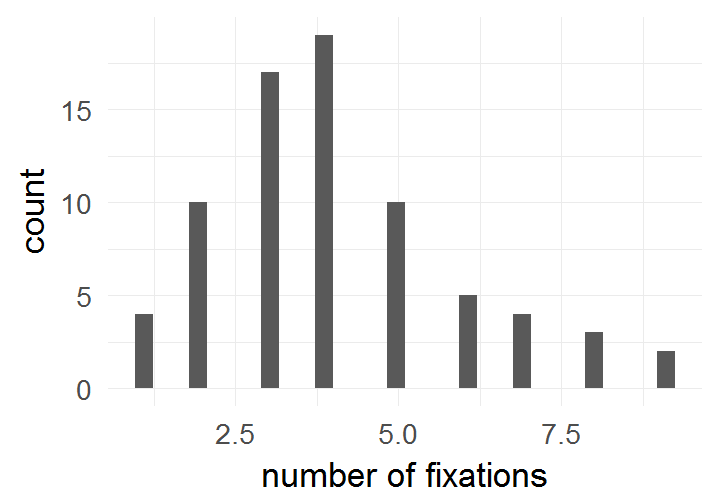### (fictional) Example: Poisson Regression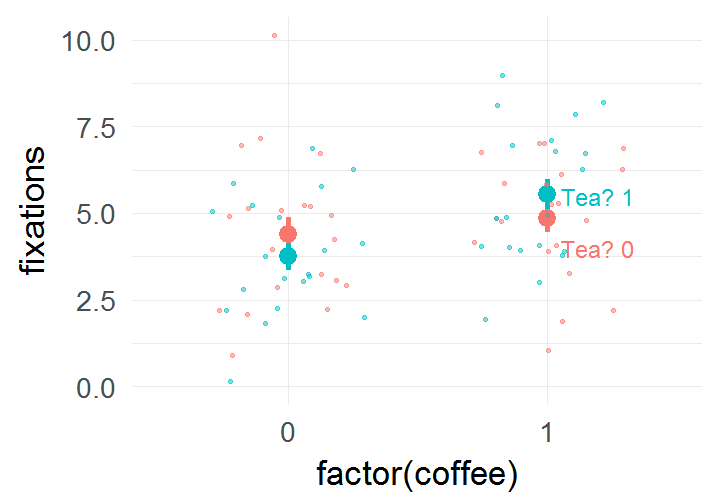### fitting in R and Interpretation


Call:
glm(formula = fixations ~ coffee * tea, family = poisson, data = df.pois)

Deviance Residuals:
Min        1Q    Median        3Q       Max
-2.73861  -0.69280   0.06776   0.59125   2.28465

Coefficients:
Estimate Std. Error z value Pr(>|z|)
(Intercept)  1.48160    0.10660  13.899   <2e-16 ***
coffee       0.09737    0.14722   0.661    0.508
tea         -0.15985    0.15715  -1.017    0.309
coffee:tea   0.29467    0.20980   1.405    0.160
---
Signif. codes:  0 '***' 0.001 '**' 0.01 '*' 0.05 '.' 0.1 ' ' 1

(Dispersion parameter for poisson family taken to be 1)

Null deviance: 75.082  on 79  degrees of freedom
Residual deviance: 67.630  on 76  degrees of freedom
AIC: 338.54

Number of Fisher Scoring iterations: 4


link-function g = $$log(\lambda) = \beta X$$

$$\lambda = e^{\beta X}$$

remember $$e^{a+b} = e^ae^b$$ => Poisson parameters are multiplicative!

### Interpretation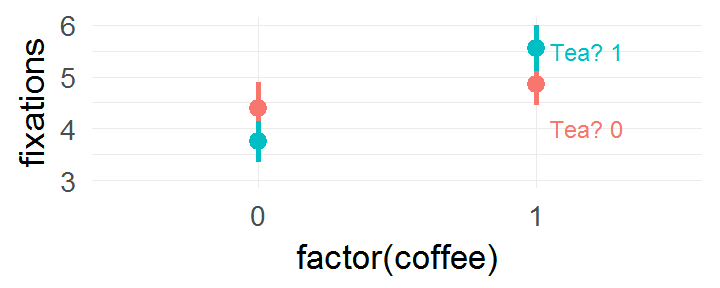(Intercept) coffee tea coffee:tea
coef(m1) 1.48 0.1 -0.16 0.29
exp(coef(m1)) 4.40 1.1 0.85 1.34
Condition $$log(\lambda) = \sum coef$$ $$\lambda = \prod e^{coef} = e^{\sum coef}$$ $$\lambda$$
Tea = 0 & Coffee = 0 1.48 4.4 4.4
Tea = 1 & Coffee = 0 1.48-0.16 4.4*0.85 3.74
Tea = 0 & Coffee = 1 1.48+0.09 4.4*1.1 4.84
Tea = 1 & Coffee = 1 1.48-0.16+0.09+0.29 4.4*0.85*1.1 5.51
anova(m1,test = 'LRT')

Analysis of Deviance Table

Model: poisson, link: log

Response: fixations

Terms added sequentially (first to last)

Df Deviance Resid. Df Resid. Dev Pr(>Chi)
NULL                          79     75.082
coffee      1   5.4717        78     69.611  0.01933 *
tea         1   0.0027        77     69.608  0.95859
coffee:tea  1   1.9782        76     67.630  0.15958
---
Signif. codes:  0 '***' 0.001 '**' 0.01 '*' 0.05 '.' 0.1 ' ' 1

exp(coef(m1)) # what are the rates?

(Intercept)      coffee         tea  coffee:tea
4.4000000   1.1022727   0.8522727   1.3426804


link-function g = $$log(\lambda)$$

### Overview

• Binary data
• Example
• Logistic regression
• Interpretation of coefficients
• Checking the model
• Inference
• Poisson Regression
• GLMs
• Assumptions

### The GLM family

Probability Distribution Link-Function (g) Statistical name
Normal $$\beta X = y$$ Linear Regression
Bernoulli $$\beta X = log(\frac {p} {1-p})$$ Logistic Regression
Poisson $$\beta X = log(\lambda)$$ Poisson Regression
ExponentialGamma $$\beta X = \frac 1 x$$ Gamma Regression

### Overview

• Binary data
• Example
• Logistic regression
• Interpretation of coefficients
• Checking the model
• Inference
• Poisson Regression
• GLMs
• Assumptions

### Assumptions

• all GLMs data points are independent
• the variance follows the mean in a specific way:
• Binomial: $$\sigma^2 = \mu(1-\mu)$$
• Poisson: $$\sigma^2 = \mu$$
• Gamma: $$\sigma^2 = \mu^2$$
• Normal: $$\sigma^2 = 1$$ (constant)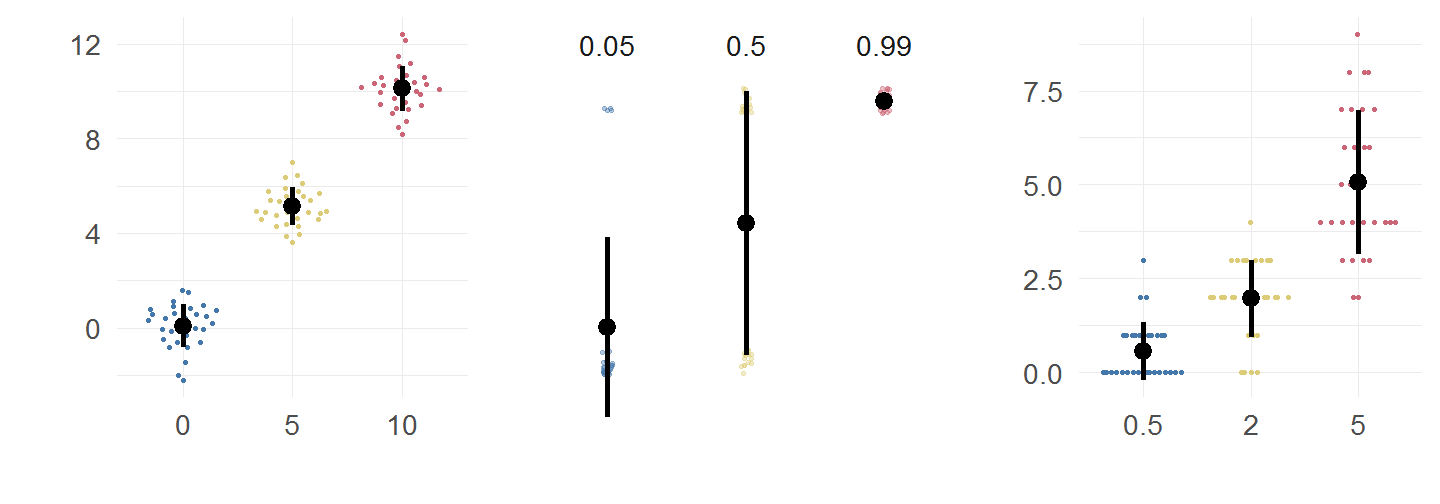### Poisson: A simple example when var != mean

dataSubset = df.pois$coffee==0 & df.pois$tea==0
mean(df.pois$fixations[dataSubset])   4.4  var (df.pois$fixations[dataSubset])

 4.778947

mean(df.pois$fixations[dataSubset]*2)   8.8  var (df.pois$fixations[dataSubset]*2)

 19.11579


### Binomial: It is more complicated

Impossible to have under/overdispersion with only an intercept

$$\sigma^2 = \mu(1-\mu)$$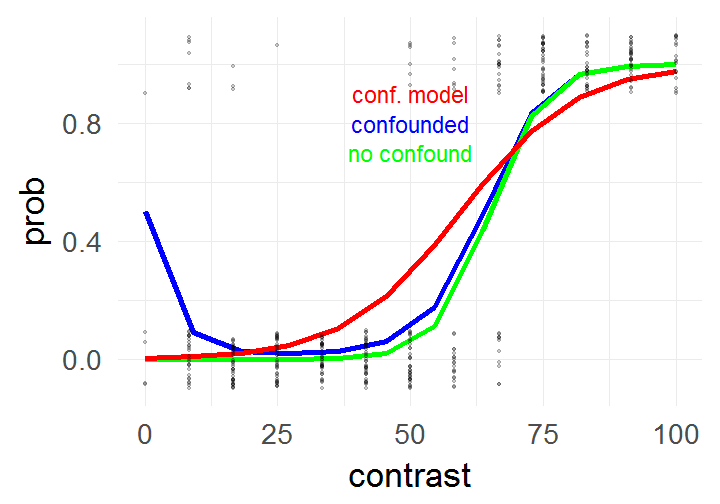# Calculate dispersion parameter
sum(resid(mres, type = "pearson")^2) / df.residual(mres) # should be 1

 3.384014


### deviance residual plot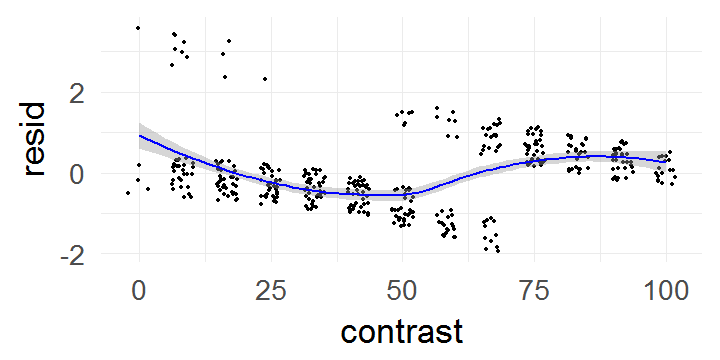In the case of the confound a non-linearity can be seen.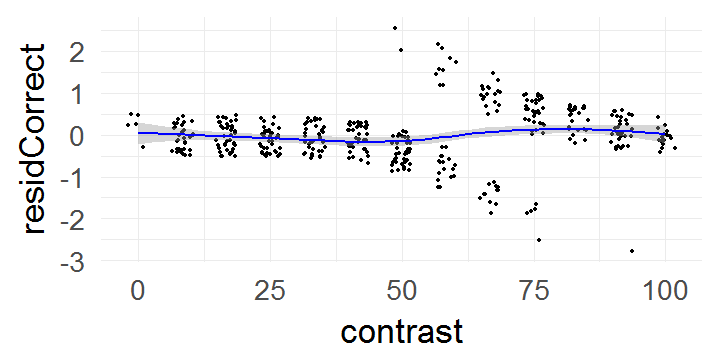This is absent in the data without the confound

### What to do?

Under/Overdispersion can occur in all GLMs (except normal)

### Overdispersion comes mostly from:

• Missing Factors
• Non-linear effects
• wrong link function
• outliers

### How to find out?

• Check relation between variance and mean
• logistic: Dispersion parameter read more
• Poisson: mean / variance for binned data

### How to compensate

• Difficult in general
• Quasibinomial / quasipoisson
• Negative binomial read more)
• Check out DHARMa (read more)

### Quasibinomial

Estimate an additional variance scaling factor. Thus (e.g. for Poisson):

$Var = \phi \cdot mean$

  mres1 = glm(data=d,response~ 1 + contrast,family=binomial)

 "             Estimate Std. Error z value Pr(>|z|)    "
 "(Intercept) -5.514545   0.522216  -10.56   <2e-16 ***"
 "contrast     0.092564   0.008715   10.62   <2e-16 ***"
 "(Dispersion parameter for binomial family taken to be 1)"

sum(resid(mres1, type = "pearson")^2) / df.residual(mres1)

 3.384014

mres2 = glm(data=d,response~ 1 + contrast,family=quasibinomial)

 "            Estimate Std. Error t value Pr(>|t|)    "
 "(Intercept) -5.51455    0.96065  -5.740 1.88e-08 ***"
 "contrast     0.09256    0.01603   5.774 1.56e-08 ***"
 "(Dispersion parameter for quasibinomial family taken to be 3.384015)"


Quasi-likelihood makes model comparisons difficult!

### Summary

• $$\hat y =$$ g $$(\beta X)$$
• choose link function appropriately
• remember that estimated parameters are on a non-linear scale
• check your assumptions

### (ignore this slide) load JS-scripts

cat("

<script type='text/javascript' src='C:/Program Files/RStudio/resources/presentation/revealjs/plugin/notes/notes.js'></script>
")